# RD Sharma Solutions - Chapter 18 - Practical Geometry (Constructions) (Part - 2), Class 8, Maths Notes | Study RD Sharma Solutions for Class 8 Mathematics - Class 8

## Class 8: RD Sharma Solutions - Chapter 18 - Practical Geometry (Constructions) (Part - 2), Class 8, Maths Notes | Study RD Sharma Solutions for Class 8 Mathematics - Class 8

The document RD Sharma Solutions - Chapter 18 - Practical Geometry (Constructions) (Part - 2), Class 8, Maths Notes | Study RD Sharma Solutions for Class 8 Mathematics - Class 8 is a part of the Class 8 Course RD Sharma Solutions for Class 8 Mathematics.
All you need of Class 8 at this link: Class 8

PAGE NO 18.13:

Question 1:

Construct a quadrilateral ABCD given that AB  = 4 cm, BC  = 3 cm, ∠A  = 75°, ∠B  = 80° and ∠C  = 120°.

Steps of construction:

Step I: Draw AB = 4 cm.

Step II: Construct ∠XAB = 75° at A and ∠ABY = 80° at B.

Step III: With B as the centre and radius 3 cm, cut off BC = 3 cm.

Step IV: At C, draw ∠BCZ = 120° such that it meets AX at D.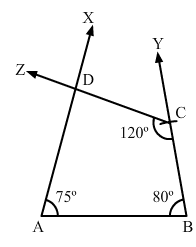Question 2:

Construct a quadrilateral ABCD, where AB  = 5.5 cm, BC  = 3.7 cm, ∠A  = 60°, ∠B  = 105° and ∠D  = 90°.

We know that the sum of all the angles in a quadrilateral is 360.

i.e., ∠A + ∠B + ∠C + ∠D = 360°

⇒ ∠C = 105°

Steps of construction:

Step I: Draw AB = 5.5 cm.

Step II: Construct ∠XAB = 60° at A and ∠ABY = 105°.

Step III: With B as the centre and radius 3.7 cm, cut off BC = 3.7 cm.

Step IV: At C, draw ∠BCZ = 105° such that it meets AX at D.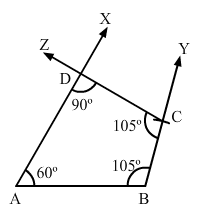Question 3:

Construct a quadrilateral PQRS, where PQ  = 3.5 cm, QR  = 6.5 cm, ∠P  = ∠R  = 105° and ∠S  = 75°.

We know that the sum of all the angles in a quadrilateral is 360.

i.e., ∠P + ∠Q + ∠R + ∠S = 360°⇒ ∠Q = 75°

Steps of construction:

Step I: Draw PQ = 3.5 cm.

Step II: Construct ∠XPQ = 105° at P and ∠PQY = 75° at Q.

Step III: With Q as the centre and radius 6.5 cm, cut off QR = 6.5

Step IV: At R, draw ∠QRZ = 105° such that it meets PX at S.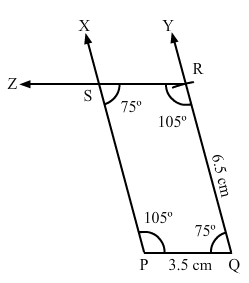Question 4:

Construct a quadrilateral ABCD when BC  = 5.5 cm, CD  = 4.1 cm, ∠A  = 70°, ∠B  = 110° and ∠D  = 85°.

We know that the sum of all the angles in a quadrilateral is 360.

i.e., ∠A + ∠B + ∠C + ∠D = 360°

⇒ ∠C = 95°

Steps of construction:

Step I: Draw BC = 5.5 cm.

Step II: Construct ∠XBC = 110° at A and ∠BCY = 95°.

Step III: With C as the centre and radius 4.1 cm, cut off CD = 4.1 cm.

Step IV: At D, draw ∠CDZ = 85° such that it meets BY at A.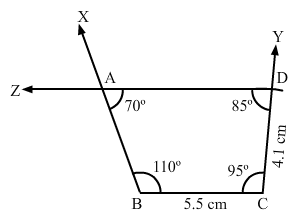Question 5:

Construct a quadrilateral ABCD, where ∠A  = 65°, ∠B  = 105°, ∠C  = 75° BC  = 5.7 cm and CD  = 6.8 cm.

We know that the sum of all the angles in a quadrilateral is 360.

i.e., ∠A + ∠B + ∠C + ∠D = 360°

⇒ ∠D = 115°

Steps of Construction:

Step I: Draw BC = 5.7 cm.

Step II: Construct ∠XBC = 105° at B and ∠BCY = 105° at C.

Step III : With C as the centre and radius 6.8 cm, cut off CD = 6.8 cm.

Step IV: At D, draw ∠CDZ = 115° such that it meets BY at A.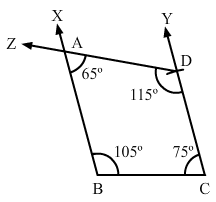Question 6:

Construct a quadrilateral PQRS, in which PQ  = 4 cm, QR  = 5 cm, ∠P  = 50°, ∠Q  = 110° and ∠R  = 70°.

Steps of construction:

Step I: Draw PQ = 4 cm.

Step II: Construct ∠XPQ = 50° at P and ∠PQY = 110° at Q.

Step III : With Q as the centre and radius 5 cm, cut off QR = 5 cm.

Step IV: At R, draw ∠QRZ = 70° such that it meets PX at S.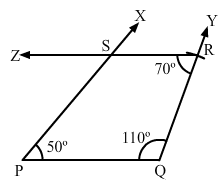The document RD Sharma Solutions - Chapter 18 - Practical Geometry (Constructions) (Part - 2), Class 8, Maths Notes | Study RD Sharma Solutions for Class 8 Mathematics - Class 8 is a part of the Class 8 Course RD Sharma Solutions for Class 8 Mathematics.
All you need of Class 8 at this link: Class 8Use Code STAYHOME200 and get INR 200 additional OFF

## RD Sharma Solutions for Class 8 Mathematics

88 docs

Track your progress, build streaks, highlight & save important lessons and more!

,

,

,

,

,

,

,

,

,

,

,

,

,

,

,

,

,

,

,

,

,

,

,

,

,

,

,

;